Next: Exponentiation: xy Up: Logarithmic Functions Previous: Exponential Function

### Natural Logarithm Function

Computing the natural logarithm function is performed using the approximation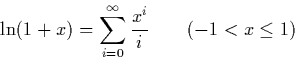Using this we can construct a sequence S(x) with the property that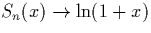as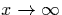as follows: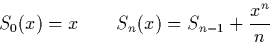We can prove that if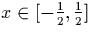, this converges to the limit with a rate of convergence which ensures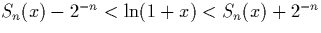. In order to get an upper and lower bound on the value of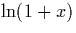, we need only take the first n digits of the (corrected to take account of the exponent), and cons' this list onto an infinite sequence of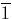's for the lower bound or of 1's for the upper bound.

This allows us to computegiven that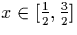. More generally, however, we would like to be able to compute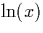for all values of (x > 0). In order to do this, we use the following properties of natural logarithm:

To calculate the natural logarithm of a value x, we multiply or divide x by the constant e to obtain x' which in the range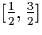. We can then apply the algorithm to compute, and use this result to work. The complicated part is that we do not have relational operators which allow us to test whether x' is in the required range (see section 2.2.2). To solve this problem we use a subtle trick which allows us to implement the algorithm using these properties.

Although we cannot actually test whether a given stream x is in the specified range, we can examine a finite portion of it and narrow the range reals it could represent until we can deduce one of the following facts: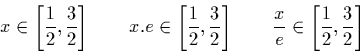We then repeatedly multiply or divide x by e until we can observe one of these facts and proceed as before. In fact we need to examine at most five signed binary digits of x at each stage to determine whether we need to multiply or divide again or whether we can proceed to the next stage of the calculation.

At this point we can compute the limit of the sequence defined above generated by the new x after multiplication or division by e as this new x is in the required range, and use this to obtain the desired result. It is necessary to examine sufficient digits and cases to ensure that it is not possible to miss' the desired range by perpetually multiplying and dividing x by e without ever being able to definitely say that it lies in the desired range.Next: Exponentiation: xy Up: Logarithmic Functions Previous: Exponential Function
Martin Escardo
5/11/2000